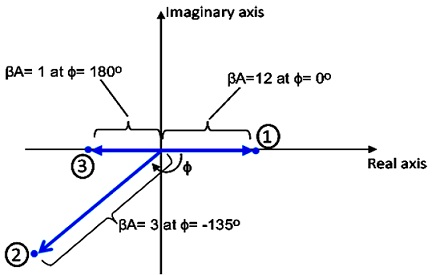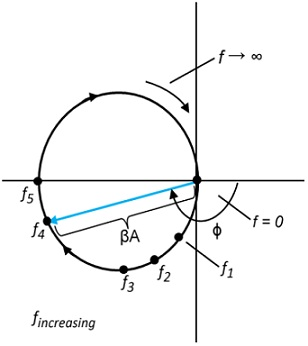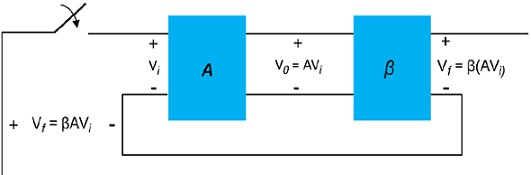#### Positive Feedback, Physics tutorial

Introduction:

If, as frequency increases, phase shift changes then some of the feedback signal will add to input signal. It is then possible for amplifier to break in oscillation because of positive feedback. If amplifier oscillates at some low or high frequency, it is no longer helpful as the amplifier. Proper feedback-amplifier design needs that circuit be stable at all frequencies, not just those in range of interest. Otherwise transient disturbance could cause seemingly stable amplifier to abruptly begin oscillating.

Nyquist Criterion:

In judging stability of the feedback amplifier, as function of frequency, βA product and phase shift between input and output are determining factors. One of the most popular methods utilized to investigate stability is Nyquist method. The Nyquist diagram is utilized to plot gain and phase shift as function of frequency on complex plane. Nyquist plot, in effect, combine two Bode plots of gain versus frequency and phase shift versus frequency and phase shift versus frequency on the single plot. The Nyquist plot is utilized to quickly show whether amplifier is stable for all frequencies and how stable amplifier is relative to some gain or phase-shift criteria.A few points of different gain (βA) values are shown at few different phase-shift angles. By using positive real axis as reference (0o), magnitude of βA = 2 is shown at phase shift of 0o at point 1. Additionally, magnitude of βA = 3 at the phase shift of -135o is shown at point 2 and magnitude/phase of βA =1 at 180o is shown at point 3. Therefore points on this plot can represent both gain magnitude of βA and phase shift. If point representing gain and phase shift for the amplifier circuit can be plotted at increasing frequency, then Nyquist plot

At origin, gain is 0 at the frequency of 0 (for RC-type of coupling). At increasing frequency, point f1, f2, and f3 and phase shift increased, as did magnitude of βA. At the representing frequency f4, value of A is vector length from origin to point f4 and phase shift is the angle. At a frequency f5, phase shift is 180o. At higher frequencies, gain is shown to decrease back to 0.Nyquist criterion for stability can be defined as follows:

• Amplifier is unstable if Nyquist curve plotted encloses (encircles) -1 point, and it is stable otherwise.

Use of positive feedback which results in feedback amplifier having closed-loop gain |Af| greater than 1 and satisfies phase condition will result in operation as the oscillator circuit. The oscillator circuit then gives varying output signal. If output signal differs sinusoidally, circuit is referred to as sinusoidal oscillator. If output voltage rises quickly to one voltage level and afterward drops rapidly to another voltage level, circuit is usually referred to as pulse or square- wave oscillator.To understand how feedback circuit executes as oscillator, consider feedback circuit. When switch at amplifier input is open, no oscillation occurs. Consider that we have fictitious voltage at amplifier input Vi. This results in the output voltage V0 after amplifier stage and in voltage Vf βAVI after feedback stage.

Therefore, we have feedback voltage Vf = β(AVi) where βA is referred to as loop gain. If circuits of base amplifier and feedback network give βA of the correct magnitude and phase, Vf can be made equal to Vi. Then, when switch is closed and fictitious voltage Vi is removed, circuit will continue operating as feedback voltage is adequate to drive amplifier and feedback circuits resulting in proper input voltage to maintain loop operation. Output waveform will still exist after switch is closed if condition βA = 1 is met. This is called as Barkhausen criterion for oscillation.

In reality, no input signal is required to start oscillator going. Only condition βA = 1 should be satisfied for self-sustained oscillations to result. In practice, βA is made greater than and system is begun oscillating by amplifying noise voltage that is always present. Saturation factors in practical circuit give average value of βA of 1. Resulting waveforms are never accurately sinusoidal. Though, the closer the value of βA is to exactly 1, the more nearly sinusoidal is waveform.

Gain and Phase Margins:

From Nyquist criterion, we know that feedback amplifier is stable if loop gain (βA) is less than unity (0 dB) when phase angle is 180o. We can additionally find out some margin of stability to point to how close to instability amplifier is. That is, if gain (βA) is less than unity but, say, 0.95 in value, this would not be as relatively stable as another amplifier having, say, (βA) = 0.7 (both measured at 180o). Of course, amplifier with loop gain 0.7 and are both stable, but one is closer to instability, if loop gain increases, than the other. We can describe the given terms:

• Gain margin (GM) is expressed as negative value of |βA| in decibels at frequency at which phase angle is 180o. Therefore, 0 dB, equal to value of βA = 1, is on border of stability and any negative decibel value is stable.
• Phase margin (PM) is expressed as angle of 180o minus magnitude of angle at which value of |βA| is unity (0dB)

Tutorsglobe: A way to secure high grade in your curriculum (Online Tutoring)

Expand your confidence, grow study skills and improve your grades.

Since 2009, Tutorsglobe has proactively helped millions of students to get better grades in school, college or university and score well in competitive tests with live, one-on-one online tutoring.

Using an advanced developed tutoring system providing little or no wait time, the students are connected on-demand with a tutor at www.tutorsglobe.com. Students work one-on-one, in real-time with a tutor, communicating and studying using a virtual whiteboard technology.  Scientific and mathematical notation, symbols, geometric figures, graphing and freehand drawing can be rendered quickly and easily in the advanced whiteboard.

Free to know our price and packages for online physics tutoring. Chat with us or submit request at info@tutorsglobe.com